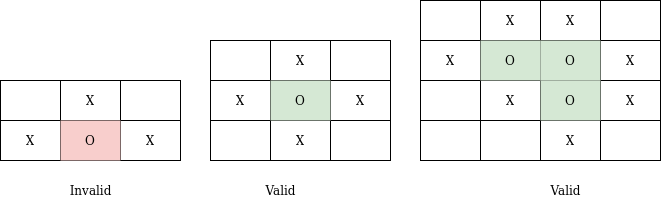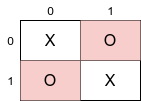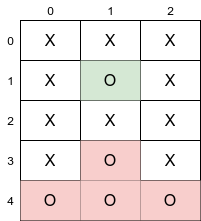3

# Capture region

Difficulty: MEDIUMContributed By
Sounak Majumder
Avg. time to solve
10 min
Success Rate
90%

Problem Statement
Suggest Edit

#### You are given a matrix having ‘N’ rows and ‘M’ columns. Each cell of the matrix is either ‘X’ or ‘O’. You need to flip all those regions of ‘O’ which are surrounded by ‘X’ i.e. you need to change all ‘O’s which are present in the region to ‘X’.

##### Note
``````1. Surrounded regions shouldn’t be on the border, which means that any 'O' on the border of the matrix is not flipped to 'X'.
2. Any ‘O’ or group of connected ‘O’ are said to be surrounded by ‘X’ when all cells touching the boundary of the group of ‘O’ must contain ‘X’.
``````
##### For example##### Input Format:
``````The first line of the input contains an integer ‘T’ denoting the number of test cases.

The next 2 * T lines describe the ‘T’ test cases.

The first line of each test case contains two space-separated positive integers ‘N’ and ‘M’ denoting the number of the rows and columns in a matrix respectively.

In the next ‘N’ lines of each test case, the ith line contains a string of length ‘M’ containing either ‘X’ or ‘O’.
``````
##### Output Format:
``````For each test case, print N lines each line containing M characters denoting the matrix element at position MATRIX[i][j] where i and j are the indices of the 2 - D array.

The output of each test case will begin from a new line.
``````
##### Note:
``````You do not need to print anything, it has already been taken care of. Just implement the given function.
``````
##### Constraints:
``````1 <= T <= 50
1 <= N <= 10 ^ 4
1 <= M <= 10 ^ 4
1 <= N * M <= 10 ^ 4

Where ‘T’ is the number of test cases, ‘N’ is the number of rows, ‘M’ is the number of columns.

Time Limit: 1 sec
``````
##### Sample Input 1:
``````2
2 2
XO
OX
5 3
XXX
XOX
XXX
XOX
OOO
``````
##### Sample Output 1:
``````XO
OX
XXX
XXX
XXX
XOX
OOO
``````
##### Explanation for sample input 1:
``````Test case 1:
The Os present at [0,1] and [1,0] both lies on boundaries hence they remain as it is.
````````````Test case 2:
The Os present at [4,0], [4,1], and [4,2] lies on boundaries hence they remain as it is.
The O present at [3,1] is connected to O present at boundaries so it will also remain the same.
The O present at [1,1] is not connected to any O which lies on the boundary so it will be considered as a captured region. Hence they will be flipped to X.
``````##### Sample Input 2:
``````2
3 3
OOO
OXO
OOO
3 3
XXX
XOX
XXX
``````
##### Sample Output 2:
``````OOO
OXO
OOO
XXX
XXX
XXX
``````Console